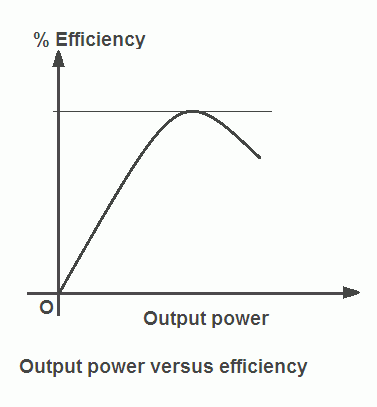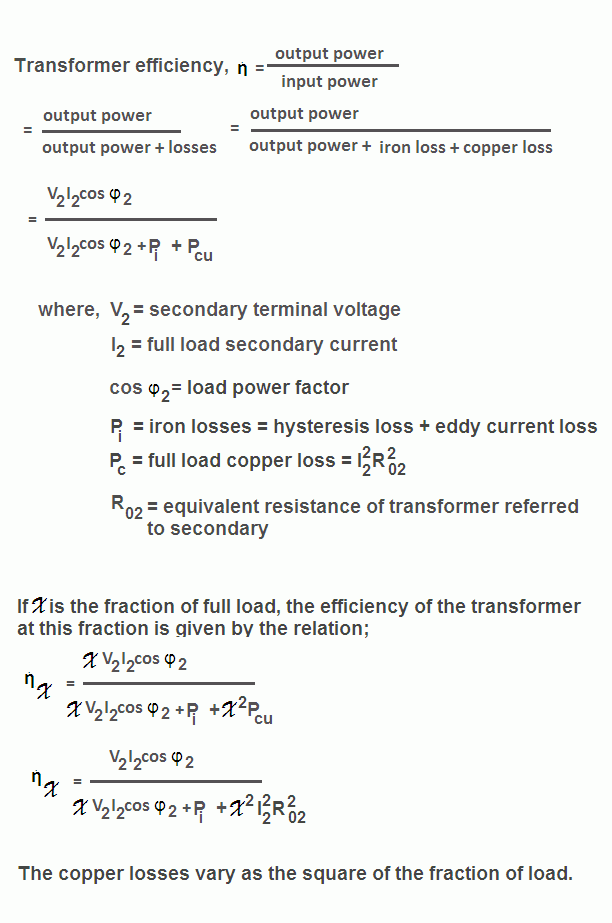# Efficiency of Transformer

The efficiency of transformer is defined as the ratio of output power to input power. It is denoted by ἠ.

As the output power is always less than the input power due to losses in the transformer, practically the transformer efficiency is always between 0 and 1 i.e. 0% and 100% but it can never be 1 or 100%.

The efficiency of an ideal transformer is equal to 1 or 100% since the losses in the ideal transformer are zero.The graph of output power versus efficiency of transformer is shown in the figure. The figure shows that the efficiency increases with the increase in the output power up to a certain value and after a particular value of output power, the transformer efficiency decreases.

The value of transformer efficiency will be maximum when the copper losses will be equal to iron losses in the transformer. The value of maximum efficiency can be found by taking total losses equal to 2Pi. It also depends on load power factor and has the maximum value at a power factor of unity.

The transformer on which load is variable (like distribution transformer) is designed to give maximum efficiency at about 75% of full load.

And if it is continuously operated near the full load (like
power transformers), then it is designed to give maximum efficiency at or near the full load.

# Efficiency of Transformer | FormulaThe transformer has no moving parts so the losses due to friction and windage are absent therefore its efficiency is very high. It can be at least equal to 90%. Its output and input are almost of the same value. Hence their ratio cannot be found accurately by measuring input and output power.

To overcome this problem it is better to measure the transformer losses separately and then find the transformer efficiency by the transformer efficiency formula. The iron losses and copper losses of can be determined very easily and accurately by no-load test and short-circuit test on transformer respectively.

## All Day Efficiency of Transformer

The efficiency discussed so far is the ordinary or commercial or power efficiency of the transformer. But for the distribution transformer, it does not give the true idea about the transformer performance because the load on distribution transformer fluctuates throughout the day. This transformer is energized for twenty-four hours, but for the major portion of the day, it delivers the very light load.

Thus iron losses take place for the whole day but copper losses take place only when the transformer is loaded.

Hence, the performance of such transformer (like distribution transformer) cannot be judged by the power efficiency. But it can be judged by the special type of transformer efficiency known as energy efficiency or all-day efficiency. The all-day efficiency is computed on the basis of energy consumed during the period of twenty-four hours.

The all day efficiency of transformer is defined as the ratio of output energy (in kWh) to input energy (in kWh) for twenty-four hours.

To find all day efficiency of transformer, we have to know the load cycle of the transformer.

## Transformer Efficiency Calculation

Example : A 500 KVA transformer has 2500 watts iron loss, and 7500 watts copper loss at full load. The power factor is 0.8 lagging. Calculate:

• transformer efficiency at full load,
• maximum efficiency of the transformer,
• output KVA corresponding to maximum efficiency,
• transformer efficiency at half load.

Solution: Transformer rating = 500 KVA
Transformer output power = 500,000 x 0.8 = 400,000 watts

Iron losses (Pi) = 2500 W
Full load copper loss (Pcu) = 7500 W

## Transformer Efficiency at Full Load

= [(output power)/(output power + Pi +Pcu)] x 100

= [(400,000)/(400,000 + 2500 + 7500)] x 100

= 97.56% (Ans)

## Maximum Efficiency of Transformer

For maximum efficiency, Copper loss (Pc) = Iron losses (Pi) = 2500 W

= [(output power)/(output power + Pi +Pc)] x 100

Therefore, maximum efficiency = [(400,000)/(400,000 + 2500 + 2500)] x 100

= 98.76% (Ans)

## Output KVA Corresponding to Maximum Efficiency

= full load KVA x √(Pi/Pc)

= 500 x √(2500/7500)
= 500 x √0.333 = 166.5 KVA (Ans)

## Transformer Efficiency at Half Load

Fraction of load at which efficiency is to be calculated (x) = half load = ½ = 0.5

Therefore, x = 0.5

Therefore, put x = 0.5 in above formula to get transformer efficiency at half load

= [(0.5 x 400,000)/{(0.5 x 400,000) + 2500 + (0.5)2 x 7500)}] x 100

= [ 200,000/ { 200,000 + 2500 + 3900 } x 100

= 96.89% (Ans)

## Calculate All-day Efficiency of Transformer

Example: A 20 KVA transformer on domestic load, which can be taken as of unity power factor, has a full load efficiency of 95.3%, the copper loss then being twice the iron loss. Calculate its ail-day efficiency at following daily cycle:

• no load for 10 hours,
• half load for 8 hours,
• full load for 6 hours.

Solution: Full load output = 20 x 1 = 20 kW
Full load input = output/efficiency = (20/95.3) x 100 = 20.986 kw

Total losses = Pi + Pcu = Input – Output = 20.986 – 20 = 0.986 kw

Now Pcu = 2Pi (given)
Therefore, Pi + 2Pi = 0.986 kW
Or Iron losses (Pi) = 0.3287 kW

Full load copper losses (Pcu) = 2 x 0.3287 = 0.6574 kW

kWh output in 24 hours = {(1/2) x 20 x 8} + (1 x 20 x 6) = 200 kWH

Iron losses for 24 hours = 0.3287 x 24 = 7.89 kW

Copper losses for 24 hours = Cu losses for 8 hours at half load + Cu losses for 6 hours at full load

= {(1/2)2 x 0.6574 x 8} + (0.6574 x 6)

= 5.259 kWH

Input in 24 hours = kWh output in 24 hours + iron and copper losses for 24 hours

= 200 + 7.89 + 5.259 = 213.149 kWH

All day efficiency of transformer = (kWH output in 24 hours/ kWH input in 24 hours) x 100

= (200/213.149) = 93.83% (Ans)

## Transformer | All Posts

© https://yourelectricalguide.com/ transformer efficiency formula & calculation.

### 2 thoughts on “Efficiency of Transformer”

1.For finding maximum efficiency of transformer,
Copper loss at full load = 7500 W
Copper loss at max efficiency condition = Iron Loss = 2500 W
Fraction of load at max efficiency condition = √(2500/7500) = (1/√3)
Transformer output power = (1/√3)*500,000 x 1 = 288675 W
Maximum efficiency = [(288675)/(288675+2500+2500)]x100
= 98.29 %